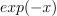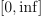/NumPy 1.17

# numpy.polynomial.laguerre.lagweight

`numpy.polynomial.laguerre.lagweight(x)` [source]

Weight function of the Laguerre polynomials.

The weight function isand the interval of integration is. The Laguerre polynomials are orthogonal, but not normalized, with respect to this weight function.

Parameters: `x : array_like` Values at which the weight function will be computed. `w : ndarray` The weight function at `x`.

#### Notes

New in version 1.7.0.

© 2005–2019 NumPy Developers
Licensed under the 3-clause BSD License.
https://docs.scipy.org/doc/numpy-1.17.0/reference/generated/numpy.polynomial.laguerre.lagweight.html